Fixed Points and Fair Divisions
Berkeley Math Circle, November 11, 2001
Kiran S. Kedlaya (Berkeley)
Caution: these notes were originally written for graduate students, so you may find them tough reading at first. Hopefully this will be a lot less true by the end of the talk! What do these two facts have in common?
(a)
If one copy of a map is crumpled up and placed on top of a second, uncrumpled copy of the map, then there is a location whose image on the crumpled map lies directly over its image on the uncrumpled map.
(b)
Any group of people can divide a cake amongst themselves so that each person believes her piece is the biggest.
The former is of course the Brouwer Fixed-Point Theorem (in two dimensions), while the latter is known to some economists as the Fair Division Theorem (or more precisely, the Envy-Free Fair Division Theorem). The overt purpose of this talk is to illustrate the close relationship between these two results, and in particular their common genesis from a combinatorial result known as Sperner's Lemma. The secret purpose is to motivate people to think more about this connection, since at present it lacks a certain naturality'' that I think is hidden somewhere, and ought to be brought to the fore. Advertisement: the entire contents of this talk are shamelessly lifted from the article Rental Harmony: Sperner's Lemma in Fair Division'' by Francis Su, in the December 1999 American Mathematical Monthly, pp. 930-942. You can play around with the fair division algorithms in this talk using a Java applet at his web site:
http://www.math.hmc.edu/~su/fairdivision/
Before proceeding, let us fix some definitions. The simplex in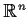is the set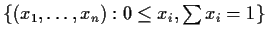. We number the vertices so that vertex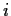is the one with nonzero-th coordinate. The facets of this simplex are its intersections with its supporting hyperplanes; a codimension 1 facet is also called a face. We number the faces so faceis opposite vertex. A triangulation of the simplex is a covering of the simplex with subsimplices, such that if two subsimplices intersect, their intersection is a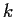-facet of each of the two, for some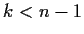.

Lemma 1 (Sperner's Lemma)   Consider a triangulation of the simplex in, whose subvertices are each labeled with one of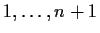, subject to the restriction that each subvertex lying on the facet of the simplex with vertices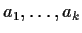is itself labeled with one of. Then the number of subsimplices whose subvertices are labeledis odd and thus nonzero. (If counted with orientation, the number is equal to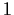.)

We defer the proof for the time being. For now, let us see how this lemma implies both the Fixed Point Theorem and the Fair Division Theorem.

Theorem 1 (Fixed Point Theorem)   Any continuous map from the simplex into itself has a fixed point.

Proof. [Proof of the Fixed Point Theorem.] For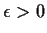, construct a triangulation such that no two points within a subsimplex have any coordinate differing by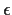or greater, or map to points with any coordinate differing byor greater. Label each subvertex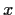with a numbersuch that the-th coordinate ofis nonzero, and is greater than or equal to the-th coordinate of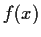. This labeling is a Sperner labeling: on the facet with vertices, only these coordinates are nonzero. Thus there exists a subsimplex labeled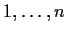. No point in this subsimplex can move to a point with a coordinate increased by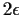or more, since there is a point in the simplex for which this coordinate decreases. By compactness, there is a sequence oftending to 0 whose corresponding subsimplices have nonempty intersection. Any point in this intersection must map to itself (since none of its coordinates can increase by more thanfor arbitrarily small).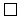Theorem 2 (Envy-Free Fair Division Theorem)   Consider a one-dimensional cake to be divided into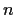pieces by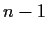cuts. From each possible division, each ofplayers designates one or more pieces as preferred''. These preferences obey the following rules:
(a)
Continuity: If a player prefers a piece in each of a set of divisions, he prefers that piece in each limiting division.
(b)
Boundary condition: No player ever prefers an empty piece.
Then there exists a division and an assignment of each piece to a different player, such that each player receives one of his preferred pieces.

Beware that the well-known moving-knife'' method does not suffice to prove this result. In that method, a knife is drawn sideways across the cake; as soon as a player believes the knife has covered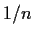of the cake, he signals the knife-mover to cut and takes the resulting piece. The remaining players then repeat the procedure until each player receives a piece. This method guarantees that each player receives at leastof the cake in his own measure (since he believes the pieces cut off before the one he receives are smaller than, so even if he gets the last piece, it will have size at least). There are two reasons why our result is stronger than that given by the moving-knife method. First, our theorem does not assume that players' preferences are given by measures on the cake. For example, if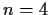, a player could prefer piece 1 in one division, and piece 2 in a division that differs from the first only in the sizes of pieces 3 and 4! Second, even if the preferences are given by measures, the moving-knife method only guarantees that a player receives a piece as least as large as the average piece, and not necessarily the biggest piece (this is why our theorem is called envy-free'').

Proof. [Proof of the Fair Division Theorem] (due to Forest Simmons) Identify the possible divisions with the simplex in, so that vertexcorresponds to all of the cake lying in piece. For, construct a triangulation of the simplex such that no two subvertices are at distanceor greater, and which can be labeled by the names of the players so that each subsimplex has subvertices labeled by the names of all of the players. (Given a triangulation satisfying the first condition, its barycentric subdivision satisfies both conditions: for each of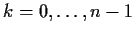, label all of the barycenters of-simplices with a single player.) Now construct a second labeling of this triangulation as follows: for each subvertex, query the player whose name labels that subvertex, and label the subvertex with one of his preferred pieces in the corresponding division. The result is a Sperner labeling, since on the facet with vertices, only piecesare nonempty, so only they will be preferred. Thus there exists a subsimplex labeled, which is to say, a subsimplex where each player chooses a different piece at his corresponding subvertex. By compactness (and the finiteness of the number of ways for the players to choose pieces), there exists a sequence oftending to 0 such that the corresponding subsimplices have nonempty intersection, and such that each player chooses the same piece each time his name occurs as the label of a subvertex. A point in that intersection is a division in which each player prefers a different piece, as desired.Proof. [Proof of Sperner's Lemma] We proceed by induction on. By the induction hypothesis, the face with vertices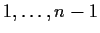has been given a Sperner labeling and so contains an odd number of subsimplices labeled. We refer to each subface labeledas a door. We have just seen that there are an odd number of doors on the boundary of the simplex. Moreover, each subsimplex contains 1 door if it is labeled, and 0 or 2 doors otherwise. Now consider the graph on the subsimplices, which are considered adjacent if they share a common subface which is also a door. This graph is a disjoint set of paths of two types: those starting and ending on the boundary, and those starting on the boundary and ending at a subsimplex labeled. Since each path of the former type uses two boundary doors, there are an odd number of the second type, and so an odd number of subsimplices labeled.The algorithmic nature of this proof yields approximate algorithms for finding fixed points and fair divisions. The Fair Division Theorem concerns division of benefits. What about the dual situation, where players must divide a certain cost? For example, ifgraduate students share an apartment, and decide to divide the rent by room, can this division be set so that each person will opt for a different room? As we shall see, Sperner's lemma, or rather a dual incarnation of it, can answer this question as well.

Theorem 3 (Rental Division Theorem)   Considerrooms and a total rent to be divided among the rooms. For each such division, each ofplayers designates one or more rooms as preferred''. These preferences obey the following rules:
(a)
Continuity: If a player prefers a piece in each of a set of divisions, he prefers that piece in each limiting division.
(b)
Boundary condition: If there is a room with rent at most 0, no player will prefer a room with positive rent.
Then there exists a division and an assignment of each room to a different player, such that each player receives one of his preferred rooms.

Lemma 2 (Dual Sperner's Lemma)   Consider a triangulation of the simplex in, whose subvertices are each labeled with one of, subject to the restriction that each subvertex lying on the intersection of facesis itself labeled with one of. Assume moreover that each facet contains a subvertex in its interior. Then the number of subsimplices whose vertices are labeledis odd and thus nonzero.

Proof. [Proof of Dual Sperner's Lemma] As in the proof of Sperner's Lemma, it suffices to show that the number of doors on the boundary of the simplex is odd. Note that a door must have at least one subvertex on each of faces. This restriction, together with the condition that the door lies on the boundary, forces the vertex at the intersection of facesto be a subvertex of the door. Without loss of generality suppose this vertex is labeled. Then of the other subvertices of the door, one must lie on each of face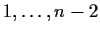, and so the door must have a second subvertex on the intersection of face. Repeating this argument shows that in fact there is exactly one door on the boundary of the simplex. Thus there are an odd number of subsimplices labeledby the same argument as above.Proof. [Proof of the Rental Division Theorem] Identify the possible divisions with the simplex in, so that the-th coordinate represents the price of room, triangulated as in the proof of the Fair Division Theorem. For, construct a triangulation of the simplex such that no two subvertices are at distanceor greater, and which can be labeled by the names of the players so that each subsimplex is labeled with the names of all of the players. Now construct a second labeling of this triangulation as follows: for each subvertex, query the player whose name labels that subvertex, and label the subvertex with one of that player's preferred rooms in the corresponding division. This new labeling is a dual Sperner labeling: on the intersection of facets, roomshave zero rent and so no other rooms will be preferred. Thus the Dual Sperner's Lemma implies the existence of a subsimplex labeled. The remainder of the argument is the same as that of the Fair Division Theorem.In conclusion, it should be noted that other results from topology also yield fair division results, and more specifically results in combinatorial topology yield approximate algorithms for fair division problems. For example, the Borsuk-Ulam theorem (any map from the-sphere tomaps some pair of antipodal points to the same point) yields the fact that a cake can be divided in two so that each ofpeople prefers both pieces equally. (The proof of the latter is similar to that of the Ham Sandwich Theorem.) On the other hand, the correspondence between topology and fair division is not completely understood; we have fragmentary results, but no unifying treatment.